# 1039. Minimum Score Triangulation of Polygon

## Problem Setting¶

Given N, consider a convex N-sided polygon with vertices labelled A, A[i], ..., A[N-1] in clockwise order.

Suppose you triangulate the polygon into N-2 triangles. For each triangle, the value of that triangle is the product of the labels of the vertices, and the total score of the triangulation is the sum of these values over all N-2 triangles in the triangulation.

Return the smallest possible total score that you can achieve with some triangulation of the polygon.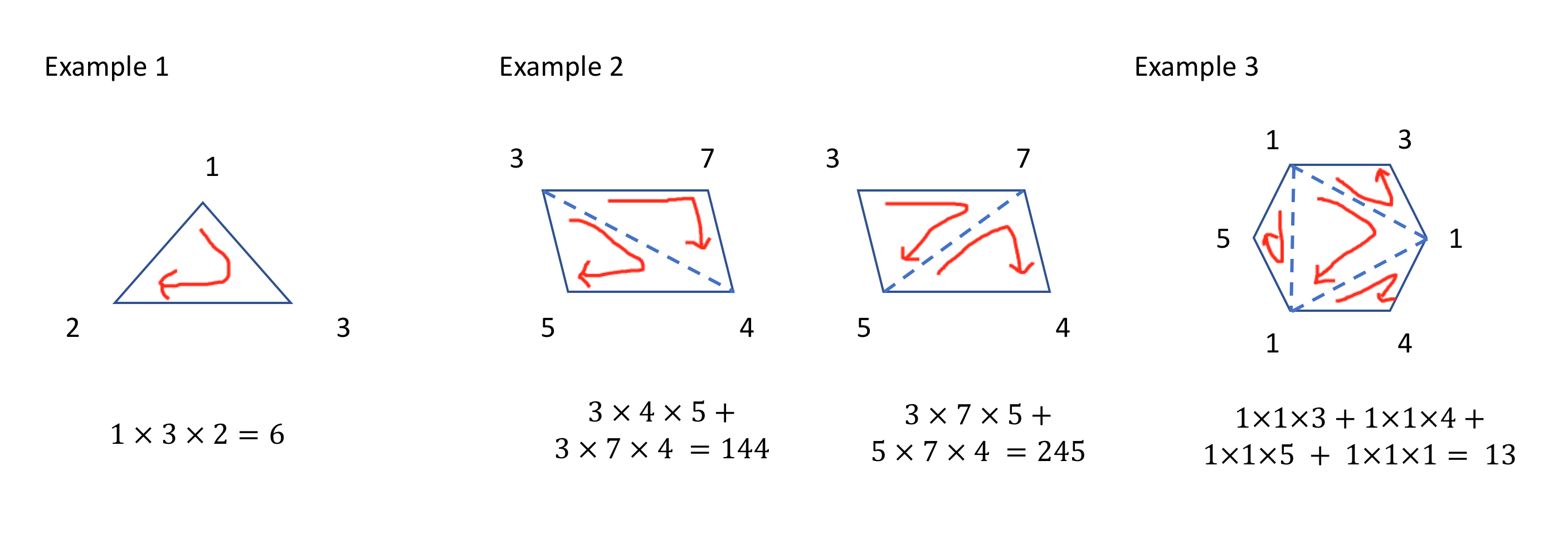The solution is based on dynamic programming like below. This gif is explained in the last part.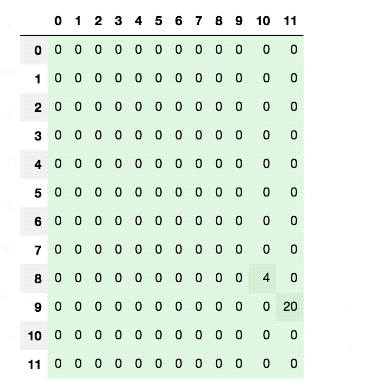### Example 1¶

Input: [1,2,3]

Output: 6

Explanation: The polygon is already triangulated, and the score of the only triangle is 6.

### Example 2¶

Input: [3,7,4,5]

Output: 144

Explanation: There are two triangulations, with possible scores: 375 + 457 = 245, or 345 + 347 = 144. The minimum score is 144.

### Example 3¶

Input: [1,3,1,4,1,5]

Output: 13

Explanation: The minimum score triangulation has score 1 1 3 + 1 1 4 + 1 1 5 + 1 1 1 = 13.

## Solution from Laurant¶

In :
from typing import List
import math
class Solution:
def minScoreTriangulation(self, A: List[int]) -> int:
n = len(A)
a = A + A
f = [ * (n + n) for i in range(n + n)]
for i in range(n + n, -1, -1):
for j in range(i + 2, n + n):
f[i][j] = math.inf
for k in range(i + 1, j):
f[i][j] = min(f[i][j], f[i][k] + f[k][j] + a[i] * a[j] * a[k])
return f[n - 1]


In :
# execute
A = [1,3,1,4,1,5]
s = Solution()
s.minScoreTriangulation(A)

Out:
13

### Step-by-step understanding¶

This problem can be solved by using dynamic programming. Overall flow is as blew:

1. Initial setting
2. Expand a list A to a list A + A
3. Move 2 points (i, j)
4. Move 3rd pointers k which divides the polygon specified by (i, j)
5. Calculate minimum score of triangle multiplication
6. Return the score when (i, j)=(0, n-1), which is the original polygon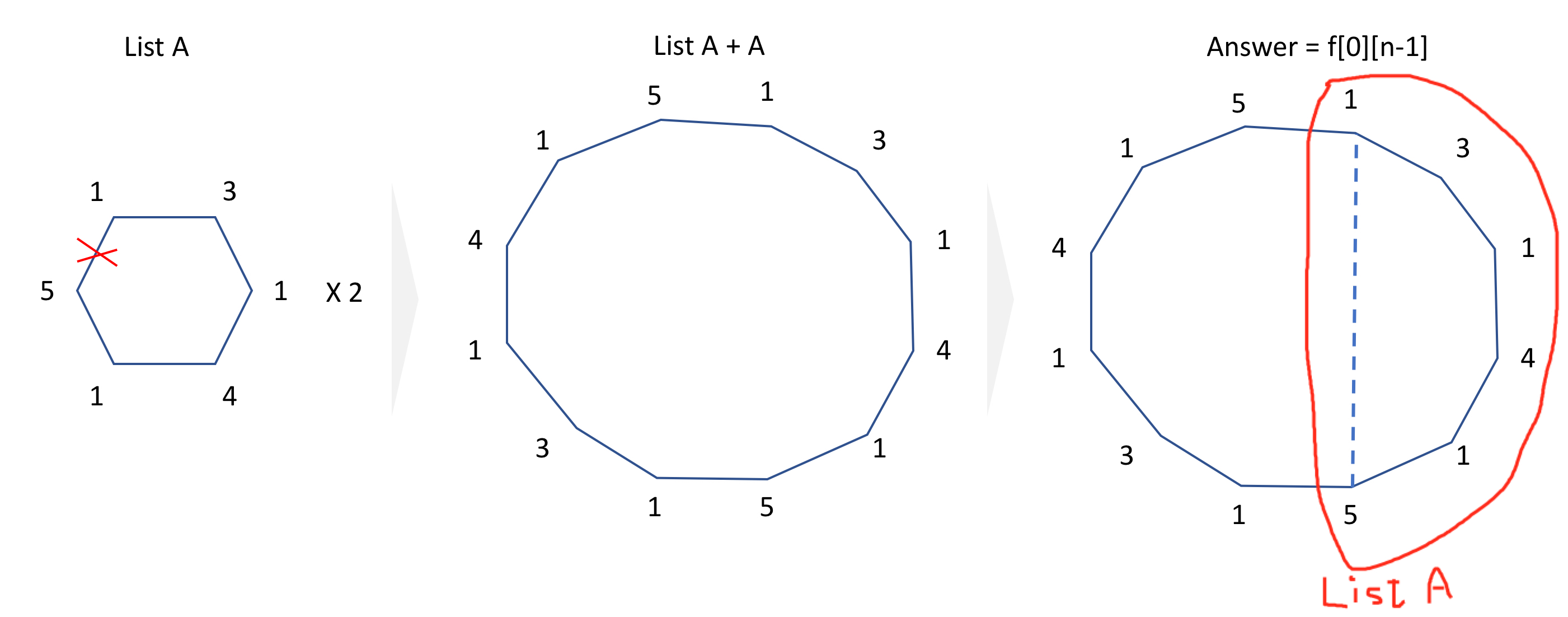#### Initial setting¶

There are three variables created:

• n: length of a list A
• a: A + A
• f: 2n by 2n zeros matrix
In :
n = len(A)
a = A + A
f = [ * (n + n) for i in range(n + n)]
print(f'n: {n}\na: {a}\n')
print(f'f: ')
f

n: 6
a: [1, 3, 1, 4, 1, 5, 1, 3, 1, 4, 1, 5]

f:

Out:
[[0, 0, 0, 0, 0, 0, 0, 0, 0, 0, 0, 0],
[0, 0, 0, 0, 0, 0, 0, 0, 0, 0, 0, 0],
[0, 0, 0, 0, 0, 0, 0, 0, 0, 0, 0, 0],
[0, 0, 0, 0, 0, 0, 0, 0, 0, 0, 0, 0],
[0, 0, 0, 0, 0, 0, 0, 0, 0, 0, 0, 0],
[0, 0, 0, 0, 0, 0, 0, 0, 0, 0, 0, 0],
[0, 0, 0, 0, 0, 0, 0, 0, 0, 0, 0, 0],
[0, 0, 0, 0, 0, 0, 0, 0, 0, 0, 0, 0],
[0, 0, 0, 0, 0, 0, 0, 0, 0, 0, 0, 0],
[0, 0, 0, 0, 0, 0, 0, 0, 0, 0, 0, 0],
[0, 0, 0, 0, 0, 0, 0, 0, 0, 0, 0, 0],
[0, 0, 0, 0, 0, 0, 0, 0, 0, 0, 0, 0]]

#### Move 2 points¶

i ranges from 2n to 0 and j ranges from i+2 to 2n

In example 3, i moves from 11 to 0 and j moves from i+2 to 11.

In :
# To visualize f
import pandas as pd
from IPython.display import clear_output, display
import seaborn as sns
cm = sns.light_palette("green", as_cmap=True)

def highlight_one(val):
"""
Takes a scalar and returns a string with
the css property 'background-color: red' for 1
, '' otherwise.
reference: https://pandas.pydata.org/pandas-docs/stable/user_guide/style.html
"""
color = 'red' if val == 1 else ''
return f'background-color: {color}'

In :
# Initialize f again
f = [ * (n + n) for i in range(n + n)]

for i in range(n + n, -1, -1):
for j in range(i + 2, n + n):
clear_output(wait=True)
f[i][j] = 1
#         display(pd.DataFrame(f).style.applymap(highlight_one))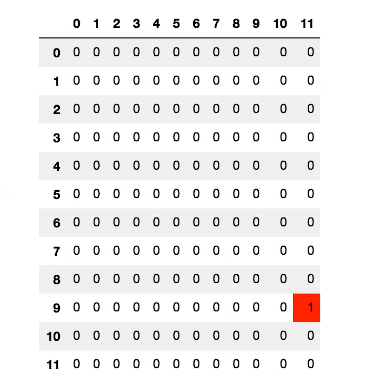#### Move 3rd pointers k which divides the polygon specified by (i, j)¶

The 3rd pointer k moves from i+1 to j. For example, when (i, j)==(7, 10), k moves 8 to 9.

for k in range(i + 1, j):
print(k)

In :
i = 7; j = 10
for k in range(i + 1, j):
print(k)

8
9


#### Calculate minimum score of triangle multiplication¶

Basically, for each (i, j), it calculates f[i][k] + f[k][j] + a[i] * a[j] * a[k] across k and keeps only the minimum values.

f[i][j] = min(f[i][j], f[i][k] + f[k][j] + a[i] * a[j] * a[k])


Let's see concrete examples when (i, j)==(7, 10), k moves 8 to 9 as mentioned above. This is the case for rectangle like example 2. k is a diagonal point.

• For k=8, f + f + a * a * a.
• For k=9, f + f + a * a * a.

When we select k, it divides the polygon specified by (i, j) into three polygons like below. Then, f[i][k], f[k][j] should be calculated already and reuse the results. The last term a[i] * a[j] * a[k] is the triangle created by k.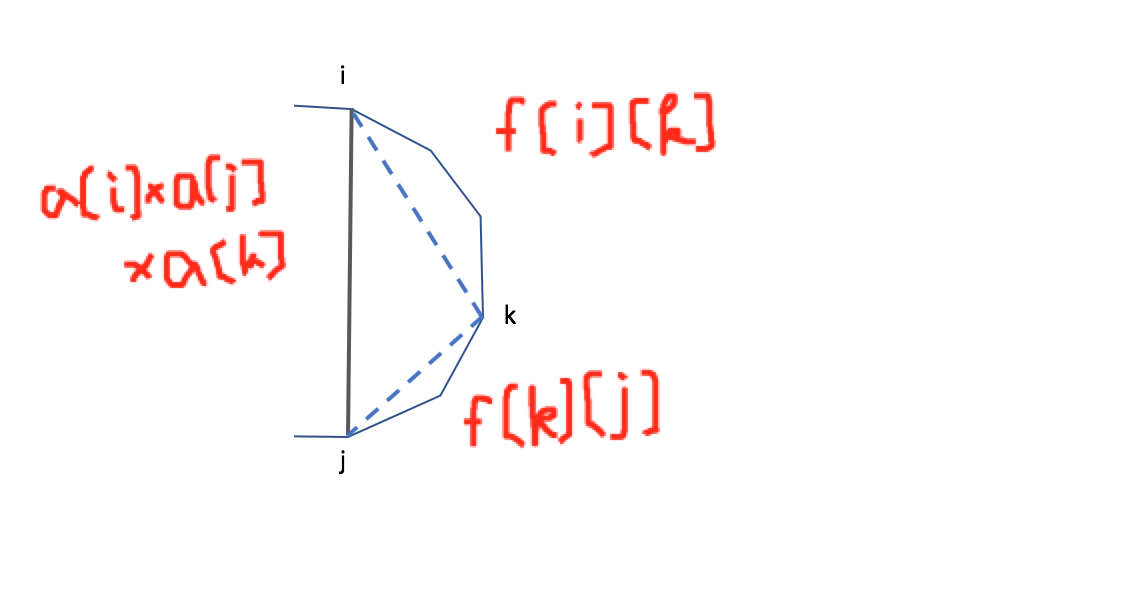In :
# Initialize f again
f = [ * (n + n) for i in range(n + n)]

for i in range(n + n, -1, -1):
for j in range(i + 2, n + n):
f[i][j] = math.inf
for k in range(i + 1, j):
clear_output(wait=True)
f[i][j] = min(f[i][j], f[i][k] + f[k][j] + a[i] * a[j] * a[k])